RRB Group D Mock Test - 35

# RRB Group D Mock Test - 35

Test Description

## 100 Questions MCQ Test RRB (Group D) - Mock Tests & Previous Year Papers | RRB Group D Mock Test - 35

RRB Group D Mock Test - 35 for Railways 2022 is part of RRB (Group D) - Mock Tests & Previous Year Papers preparation. The RRB Group D Mock Test - 35 questions and answers have been prepared according to the Railways exam syllabus.The RRB Group D Mock Test - 35 MCQs are made for Railways 2022 Exam. Find important definitions, questions, notes, meanings, examples, exercises, MCQs and online tests for RRB Group D Mock Test - 35 below.
Solutions of RRB Group D Mock Test - 35 questions in English are available as part of our RRB (Group D) - Mock Tests & Previous Year Papers for Railways & RRB Group D Mock Test - 35 solutions in Hindi for RRB (Group D) - Mock Tests & Previous Year Papers course. Download more important topics, notes, lectures and mock test series for Railways Exam by signing up for free. Attempt RRB Group D Mock Test - 35 | 100 questions in 90 minutes | Mock test for Railways preparation | Free important questions MCQ to study RRB (Group D) - Mock Tests & Previous Year Papers for Railways Exam | Download free PDF with solutions
 1 Crore+ students have signed up on EduRev. Have you?
RRB Group D Mock Test - 35 - Question 1

### Who has been recently elected as the President of Armenia?

RRB Group D Mock Test - 35 - Question 2

### Which country never reached the finals of the Cricket World Cup?

RRB Group D Mock Test - 35 - Question 3

### The Cells of Living Organisms which have a definite Nucleus are called?

RRB Group D Mock Test - 35 - Question 4

Cheruthoni dam is located in which state?

RRB Group D Mock Test - 35 - Question 5

Which of the following Hormones is generally used in medicine to treat Hypogylcemia or Low Blood Sugar?

RRB Group D Mock Test - 35 - Question 6

Where is the headquarters of Amnesty International?

RRB Group D Mock Test - 35 - Question 7

Port Louis is capital of which country?

RRB Group D Mock Test - 35 - Question 8

Which of the following is popularly called as the energy currency of the cell?

RRB Group D Mock Test - 35 - Question 9

Which of the following is not a Phase involved in Mitosis - Cell Division?

RRB Group D Mock Test - 35 - Question 10

India's first Postal Savings Office ATM is located in which city?

RRB Group D Mock Test - 35 - Question 11

Where is Salar Jung museum ?

RRB Group D Mock Test - 35 - Question 12

What is the name of the indigenous plane developed by DRDO ?

RRB Group D Mock Test - 35 - Question 13

In Bayer’s process the Bauxite one (for purification) is digested in

RRB Group D Mock Test - 35 - Question 14

Which of the following states doesn't share its border with Telangana?

RRB Group D Mock Test - 35 - Question 15

Which among the following is considered as the dance form of Kerala?

RRB Group D Mock Test - 35 - Question 16

The ratio of width to length of our National Flag is?

RRB Group D Mock Test - 35 - Question 17

What is the unit of the power of lens?

Detailed Solution for RRB Group D Mock Test - 35 - Question 17

The power of the lens is measured in diopters.

RRB Group D Mock Test - 35 - Question 18

Baking soda is also called?

RRB Group D Mock Test - 35 - Question 19

India, Bangladesh and which other country have entered into a tripartite agreement for cooperation in construction of a Nuclear Power Plant in Rooppur, Bangladesh recently?

RRB Group D Mock Test - 35 - Question 20

What is the name of FIFA 2014 Mascot ?

RRB Group D Mock Test - 35 - Question 21

Which of the following Blood Cells is also called Leukocytes?

RRB Group D Mock Test - 35 - Question 22

Jarawa tribe belongs to which State/UT of India ?

RRB Group D Mock Test - 35 - Question 23

Which of the following gland doesn’t belong to the group of Ductless Glands?

RRB Group D Mock Test - 35 - Question 24

Where is the headquarters of the ISRO?

RRB Group D Mock Test - 35 - Question 25

Kudankulam Nuclear power plant is situated in which state?

RRB Group D Mock Test - 35 - Question 26

Synapses and Dendrites are associated with

RRB Group D Mock Test - 35 - Question 27

A tissue that connects muscle to bones in humans called

RRB Group D Mock Test - 35 - Question 28

Which is the largest desert in the world?

RRB Group D Mock Test - 35 - Question 29

Shivasamudhram hydro power project is stated in which state ?

RRB Group D Mock Test - 35 - Question 30

The word ‘’Brinell’’ is associated with

RRB Group D Mock Test - 35 - Question 31

Sclerometer is used by

RRB Group D Mock Test - 35 - Question 32

Where is Atomic Power Project located in Uttar Pradesh?

RRB Group D Mock Test - 35 - Question 33

How many dance forms are there in india?

RRB Group D Mock Test - 35 - Question 34

Whenever newspapers talk about the performance of core industries, which of the following is NOT considered among them?

RRB Group D Mock Test - 35 - Question 35

Gaudiya Nritya is a dance form of which state?

RRB Group D Mock Test - 35 - Question 36

What is the name of the largest body in the asteroid belt, also classified as a dwarf planet?

RRB Group D Mock Test - 35 - Question 37

With reference to water pollution, BOD means

RRB Group D Mock Test - 35 - Question 38

What is the main component of LPG?

RRB Group D Mock Test - 35 - Question 39

D2O is also called as-

RRB Group D Mock Test - 35 - Question 40

In the context of genetics, DNA stands for

RRB Group D Mock Test - 35 - Question 41

Where is the headquarters of Central Bureau of Investigation located?

RRB Group D Mock Test - 35 - Question 42

In a multicylinder diesel engine, the cylinders are fired in a particular sequnce

RRB Group D Mock Test - 35 - Question 43

Union Ministry of Environment and Forest had approved field trials of GM food crops. In this context GM stands for

Detailed Solution for RRB Group D Mock Test - 35 - Question 43

Union Ministry of Environment and Forest Mr.M. Veerappa Moily has approved the fields trials of GM (genetically modified food crops which his predecessor Ms. Jayanti Natarajan had rejected. This move enables the seed companies and agricultural research institutes to conduct scientific field trials of different transgenic varieties of GM crops. So the answer is 2. Choice b

RRB Group D Mock Test - 35 - Question 44

Diwan-i-Khas generally refers to the place where the king ..

RRB Group D Mock Test - 35 - Question 45

Which of the following blood cells is responsible for clotting of the Blood?

RRB Group D Mock Test - 35 - Question 46

Which element group is most reactive of all the metallic elements?

RRB Group D Mock Test - 35 - Question 47

From which plant is the coffee substitute chicory made?

RRB Group D Mock Test - 35 - Question 48

Who was declared the 'Shahenshah-e-Hindustan' during the first war of Indian Independence in 1857?

RRB Group D Mock Test - 35 - Question 49

Among the following forces, which one is the weakest?

RRB Group D Mock Test - 35 - Question 50

In which city is the Leaning Tower?

RRB Group D Mock Test - 35 - Question 51

Rohan Bopanna is related to which sport ?

RRB Group D Mock Test - 35 - Question 52

What is the mathematical expression of Mass energy equation?

Detailed Solution for RRB Group D Mock Test - 35 - Question 52

ccording to the ass energy equation, E =mc2

RRB Group D Mock Test - 35 - Question 53

What is the chemical name of aspirin?

RRB Group D Mock Test - 35 - Question 54

The highest waterfalls in the world, Angel Falls, is located in which country?

RRB Group D Mock Test - 35 - Question 55

Scapula is a bone found in:

RRB Group D Mock Test - 35 - Question 56

Temple of Emerald Buddha is situated in which country?

RRB Group D Mock Test - 35 - Question 57

hich of the following schemes is not launched by the Ministry of Rural Development, Government of India?

RRB Group D Mock Test - 35 - Question 58

Durand Cup is associated with which sport?

RRB Group D Mock Test - 35 - Question 59

Escape velocity of moon is:

RRB Group D Mock Test - 35 - Question 60

Which part of brain is affected by alcohol?

RRB Group D Mock Test - 35 - Question 61

Which of the following is the least ?

Detailed Solution for RRB Group D Mock Test - 35 - Question 61

option A = 25 m/s

option B = 1560 m/min = 1560/60 m/s = 26 m/s

option C = 86.4 kmph = 86.4*(5/18) = 24 m/s

option D = 1.5 km/min = 1.5*(1000/60) = 25 m/s

So the answer is option C.

RRB Group D Mock Test - 35 - Question 62

A, B, C started a business with capital ratio 4:2:3. Their period of investiments is in the ratio 3:5:4. Find their profit ratio ?

Detailed Solution for RRB Group D Mock Test - 35 - Question 62

Ratio of their shares = (4*3):(2*5):(3*4) = 12:10:12 = 6:5:6

So the answer is option B.

RRB Group D Mock Test - 35 - Question 63

Twice a number is 12 more than half the number. Find the number ?

Detailed Solution for RRB Group D Mock Test - 35 - Question 63

2x = 12 + x/2

4x = 24 + x

3x = 24

x = 8

So the answer is option D.

RRB Group D Mock Test - 35 - Question 64

Find the LCM of 7/2, 9/4 and 5/3 ?

Detailed Solution for RRB Group D Mock Test - 35 - Question 64

LCM of fractions = LCM of numerators/HCF of denominators

LCM of 7/2, 9/4 and 5/3 = (LCM of 7,9,5)/(HCF of 2,4,3) = 315/1 = 315

So the answer is option A.

RRB Group D Mock Test - 35 - Question 65

952−52=?

Detailed Solution for RRB Group D Mock Test - 35 - Question 65

952−52=(95+5)(95−5)=100∗90=9000
So the answer is option B.

RRB Group D Mock Test - 35 - Question 66

45.23+23.17-12.46+34.67 = ?

Detailed Solution for RRB Group D Mock Test - 35 - Question 66

45.23+23.17-12.46+34.67 = 90.61

So the answer is option D.

RRB Group D Mock Test - 35 - Question 67

Convert 100111011 to its octal form ?

Detailed Solution for RRB Group D Mock Test - 35 - Question 67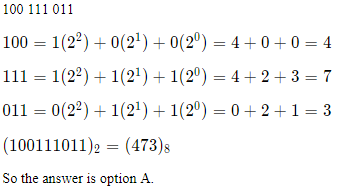RRB Group D Mock Test - 35 - Question 68

The ratio 5:4 expressed as percentage equals to ?

Detailed Solution for RRB Group D Mock Test - 35 - Question 68

5:4 = 5/4 = 1.25 = 125%

So the answer is option A.

RRB Group D Mock Test - 35 - Question 69

A merchant bought 5 stickers for a total of Rs. 10. Find the selling price of each sticker if he gains 50% ?

Detailed Solution for RRB Group D Mock Test - 35 - Question 69

Total CP = 10/-

Profit = 50% of CP = 50% of 10 = 5/-

SP = CP + profit = 10+5 = 15/-

SP of one sticker = 15/5 = 3/-

So the answer is option D.

RRB Group D Mock Test - 35 - Question 70

Ramesh invests in a scheme for two years which gives him a compound interest of 4% per annum. If he is due to receive Rs. 169 at the end of the scheme, how much should he invest today?

Detailed Solution for RRB Group D Mock Test - 35 - Question 70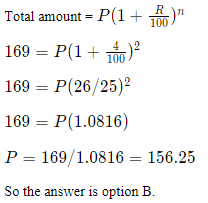RRB Group D Mock Test - 35 - Question 71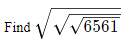Detailed Solution for RRB Group D Mock Test - 35 - Question 71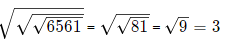So the answer is option C.

RRB Group D Mock Test - 35 - Question 72

A train takes 50 seconds to cross a bridge of length 200m.Find the length of the train if the speed of the train is 50.4kmph ?

Detailed Solution for RRB Group D Mock Test - 35 - Question 72

50.4 kmph = 50.4*(5/18) = 14 m/s

Distance = speed*time

x+200 = (14)*(50)

x+200 = 700

x = 500m

So the answer is option D.

RRB Group D Mock Test - 35 - Question 73

The average age of 4 boys is 22.5. It decreases by 6 months when a new boy is included in the group. Find the age of the new boy ?

Detailed Solution for RRB Group D Mock Test - 35 - Question 73

Total age of 4 boys = 4*22.5 = 90

Another boy of age x is added, then new average = 22.5 - 0.5 = 22

90+x = 5(22)

90+x = 110

x = 110 - 90 = 20

So the answer is option C.

RRB Group D Mock Test - 35 - Question 74

Today is Saturday, After 43 days it will be ?

Detailed Solution for RRB Group D Mock Test - 35 - Question 74

Today is Saturday.

Each day of the week is repeated after every 7 days.

Hence, after 42 days it will be Saturday.

So after 43 days, it will be Sunday.

So the answer is option C.

RRB Group D Mock Test - 35 - Question 75

If internal angle of a regular polygon is 120°, then find the number of diagonals in the polygon ?

Detailed Solution for RRB Group D Mock Test - 35 - Question 75

If internal angle of a regular polygon is 120°, it is regular hexagon,

No.of diagonals of a polygon of n sides = (n−3)/2=6(6−3)/2=6(3)/2=9

So the answer is option B.

RRB Group D Mock Test - 35 - Question 76

Find the volume of the cylinder of radius 7 cm and height 12 cm ?

Detailed Solution for RRB Group D Mock Test - 35 - Question 76

Volume of cylinder = πr2h=(22/7)(7)2(12)=1848sq.cm

So the answer is option D.

RRB Group D Mock Test - 35 - Question 77

Find the number of spheres of radius 2 cm, that can be made by melting a cube of 6 cm ?

Detailed Solution for RRB Group D Mock Test - 35 - Question 77

No.of spheres = (vol.of cube)/(vol.of sphere)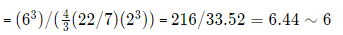So the answer is option C.

RRB Group D Mock Test - 35 - Question 78

What is the value of secθ+cosθ, if sinθ = 12/13 ?
It is known that 0 < θ < 90

Detailed Solution for RRB Group D Mock Test - 35 - Question 78

If sinθ = 12/13 cosθ = 5/13 and secθ = 13/5

secθ+cosθ = 13/5 + 5/13 = 194/65

So the answer is option A.

RRB Group D Mock Test - 35 - Question 79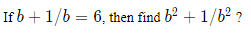Detailed Solution for RRB Group D Mock Test - 35 - Question 79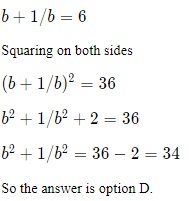RRB Group D Mock Test - 35 - Question 80

A solution of 80ltrs contains 30% acid. How many litres of pure acid should be added to this solution so that the concentration of acid becomes 65% ?

Detailed Solution for RRB Group D Mock Test - 35 - Question 80

Let x be the amount of pure acid to be added

0.3(80)+1(x) = 0.65(x+80)

24+x = 0,65x+52

x-0.65x = 52-24

0.35x = 28

x = 80

So the answer is option A.

RRB Group D Mock Test - 35 - Question 81

Select the option that is different from the other 3 options:

Detailed Solution for RRB Group D Mock Test - 35 - Question 81

Godavari, Krishna and Kaveri flow from the west to the east. Tapti flows from the east to the west. Therefore, option D is the right answer.

RRB Group D Mock Test - 35 - Question 82

Select the option that is different from the other 3 options:

Detailed Solution for RRB Group D Mock Test - 35 - Question 82

Indonesia, Philippines and Maldives are archipelago nations (consists of a group of islands). Iceland is not a archipelago. Therefore, option C is the right answer.

RRB Group D Mock Test - 35 - Question 83

Find the number which will replace ‘?’.
31, 71, 91, 32, 92, ?

Detailed Solution for RRB Group D Mock Test - 35 - Question 83

All the prime numbers from 13 are written in reverse order.
i.e 13, 17, 19, 23, 29, 31,..
are written as 31, 71, 91, 32, 92, 13

RRB Group D Mock Test - 35 - Question 84

5 friends A, B, C, D and E have 1, 2, 3, 4, and 5 coins with them not necessarily in the same order. The number of coins with B is twice the number of coins with E. C has more coins than E but fewer coins than B. A does not have the least number of coins.

Who has the highest number of coins?

Detailed Solution for RRB Group D Mock Test - 35 - Question 84

Number of coins with B = 2* Number of coins with E.
Therefore, the number of coins with B and E can be (2,1) or (4,2).
C has more coins than E but fewer coins than B. Therefore, the number of coins with B must be 4, C must be 3 and E must be 2. A does not have the least number of coins. Therefore, A must have 5 coins and D must have 1 coin.

A is the person with the highest number of coins. Therefore, option A is the right answer.

RRB Group D Mock Test - 35 - Question 85

Which of the following options must continue the series?

A, B, C, E, H, M, ?

Detailed Solution for RRB Group D Mock Test - 35 - Question 85

Let us replace the letters with their positions in the alphabetical series.

A - 1
B - 2
C - 3
E - 5
H - 8
M - 13

As we can see, the given terms are in Fibonacci series.

2+1 = 3
3+2 = 5
5+3 = 8
8+5 = 13
13+8 = 21

Therefore, the next letter must be the U, whose position in the alphabetical series is 21. Therefore, option A is the right answer.

RRB Group D Mock Test - 35 - Question 86

If SPAIN is coded as TNDES, what will be DUTCH coded as?

Detailed Solution for RRB Group D Mock Test - 35 - Question 86

S + 1 = T
P - 2 = N
A + 3 = D
I - 4 = E
N + 5 = S

Similarly,

D + 1 = E
U - 2 = S
T + 3 = W
C- 4 = Y
H + 5 = M

Therefore, option B is the right answer.

RRB Group D Mock Test - 35 - Question 87

If, ‘+’ is coded as ‘!’, ‘*’ is coded as ‘\$’, ‘-’ is coded as ‘@’, ‘/‘ is coded as ‘#’
then what is the value of 18 ! 4 \$ 7 @ 52 # 13?

Detailed Solution for RRB Group D Mock Test - 35 - Question 87

18 ! 4 \$ 7 @ 52 # 13 would mean 18 + (4*7) - (52/13) = 42
Hence, option A is right choice.

RRB Group D Mock Test - 35 - Question 88

If ‘+’ means ‘*’, ‘-’ means ‘+’, ‘*’ means ‘/’ and ‘/’ means ‘-’, then what is the value of the following expression?

119*17 + 6 / 16 * 2 + 5

Detailed Solution for RRB Group D Mock Test - 35 - Question 88

Let us replace the symbols with the actual operators.

119*17 + 6 / 16 * 2 + 5 = 119/17 * 6 - 16/2 *5

Applying BODMAS rule, we get,

119/17 * 6 - 16/2 *5 = 7*6 - 8*5
= 42 - 40
= 2

Therefore, option C is the right answer.

RRB Group D Mock Test - 35 - Question 89

According to the following venn diagram how many people play cricket, football and hockey but not kabaddi?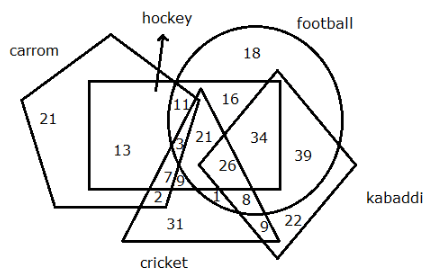Detailed Solution for RRB Group D Mock Test - 35 - Question 89

We want intersection of triangle, circle and rectangle but avoid kite.
So required value = 3 + 21 = 24
Hence, option C is the right choice.

RRB Group D Mock Test - 35 - Question 90

Identify the diagrams which best represents the relationship among the classes given below -
apple, fruit, cider

Detailed Solution for RRB Group D Mock Test - 35 - Question 90

All apples are fruit. Some cider will be made of fruits and apple.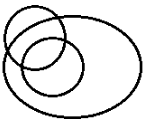Hence, option B is the right choice.

RRB Group D Mock Test - 35 - Question 91

In the question below a statement is followed by two assumptions numbered I and II. An assumption is something supposed or taken in for granted. You have to consider the statement and the following assumptions and then decide which of the assumptions is implicit in the statement.
Statement: To improve the quality of sports in India, capital expenditure in developing sports infrastructure is a must.
Assumption:
I. The quality of sports in India is not up to the mark.
II. India is short of funds to invest in developing sports infrastructure.

Detailed Solution for RRB Group D Mock Test - 35 - Question 91

Since there is a recommendation, we can assume that present quality of sports is not up to the mark. Hence, assumption I is implicit.
We cannot assume that India is short of funds and that is the reason for poor quality of sports in the country.
Hence, option A is the right answer.

RRB Group D Mock Test - 35 - Question 92

In the question below a statement is followed by two assumptions numbered I and II. An assumption is something supposed or taken in for granted. You have to consider the statement and the following assumptions and then decide which of the assumptions is implicit in the statement.
Statement: The government quickly passed a law to restrict citizens, who defaulted on their loans, to go outside the country.
Assumption:
I. There were many instances in the recent past where willful defaulters left the country.
II. It becomes difficult for the court to punish the fraudsters who are not in the country.

Detailed Solution for RRB Group D Mock Test - 35 - Question 92

Since the government was quick in passing the law, we can assume that there was an increase in the number of cases where loan defaulters left the country. Thus, assumption I is implicit.
Also, since it must be difficult for the court to punish fraudsters who are outside the country the law was passed to restrict them from leaving the country. Thus, assumption II is implicit.
Hence, option C is the right answer.

RRB Group D Mock Test - 35 - Question 93

Find the option which should replace (?), so that the second pair shares the same relation as the first pair.
QE : L :: XJ : ?

Detailed Solution for RRB Group D Mock Test - 35 - Question 93

The following relation is followed

QE : (Q(17) - E(5)) = QE : I (12)

Similarly,
XJ : (X(24) - J(10)) = XJ : N (14)

RRB Group D Mock Test - 35 - Question 94

Select the option that bears the same relationship as the words given in the question:India:
Cape of KanyaKumari :: South Africa : ?

Detailed Solution for RRB Group D Mock Test - 35 - Question 94

Cape of Kanyakumari is the southernmost tip of the Indian mainland. Similarly, Cape Agulhas is the southernmost tip of South Africa.

Therefore, option B is the right answer.

RRB Group D Mock Test - 35 - Question 95

How many children does T have?
Statement I: R is the wife of T.
Statement II: N, who is the father in law of R, has 3 grandchildren.

Detailed Solution for RRB Group D Mock Test - 35 - Question 95

From statement I, we cannot say anything about the number of children of T.
From statement II alone we do not know the relation between N, R and T.
Using statement I and II we can say that N is the father of T. But we cannot be sure that all the 3 grandchildren are children of T. It is possible that T has siblings.
Hence, option C is correct.

RRB Group D Mock Test - 35 - Question 96

If ‘WANTON’is coded as ‘AWNTNO’ and ‘SPURGE’ is coded as ‘PSRUEG’, then what is the code of ‘GENTLE’?

Detailed Solution for RRB Group D Mock Test - 35 - Question 96

The words are paired from the left and each pair is arranged in ascending order.
Thus,
WANTON = AW |NT |NO
SPURGE = PS |RU |EG

GENTEL = EG | NT | EL

RRB Group D Mock Test - 35 - Question 97

If ‘VODKA’is coded as ‘UPCLZ’ and ‘MARTINI’ is coded as ‘LBQUHOH’, then what is the code of ‘SHAKEN’?

Detailed Solution for RRB Group D Mock Test - 35 - Question 97

The odd letters are replaced with preceding letters and even letters are replaced with succeeding letters.

Thus,
VODKA will be ‘UPCLZ’
MARTINI will be ‘LBQUHOH’
‘SHAKEN’ will be ‘RIZLDO’

RRB Group D Mock Test - 35 - Question 98

If ‘RUN’ is coded as ‘53’ and ‘HUT’ is coded as ‘49’, then what is the code of ‘ANY’?

Detailed Solution for RRB Group D Mock Test - 35 - Question 98

The codes represent the numerical sum of the letters.

Thus,

RUN = R + U + N = 53

HUT = H + U + T = 49

ANY = A + N + Y = 40

RRB Group D Mock Test - 35 - Question 99

A kid cycled 8 km towards the north, then 20 km towards the east and again 4 km towards the north and finally 15 km towards east to reach the destination. What is the shortest distance between starting and ending point?

Detailed Solution for RRB Group D Mock Test - 35 - Question 99

Refer to the following figure below -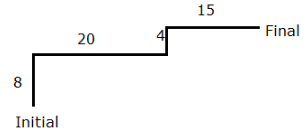The distance between final and starting point can be given by Pythagoras theorem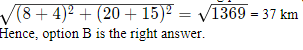RRB Group D Mock Test - 35 - Question 100

A + B means A is the brother of B
A * B means A is the mother of B
A \$ B means A is the father of B
A ÷ B means A is the husband of B
If P * Q \$ R + S ÷ T, which of the following statements is true?

Detailed Solution for RRB Group D Mock Test - 35 - Question 100

P * Q means P is the mother of Q. Q \$ R means Q is the father of R. So P is the grandmother of R. R + S means R is the brother of S. So P is also the grandmother of S.
Hence, option C is the correct option.

## RRB (Group D) - Mock Tests & Previous Year Papers

1 docs|48 tests
 Use Code STAYHOME200 and get INR 200 additional OFF Use Coupon Code
Information about RRB Group D Mock Test - 35 Page
In this test you can find the Exam questions for RRB Group D Mock Test - 35 solved & explained in the simplest way possible. Besides giving Questions and answers for RRB Group D Mock Test - 35, EduRev gives you an ample number of Online tests for practice

1 docs|48 tests